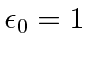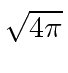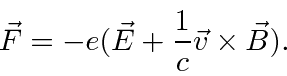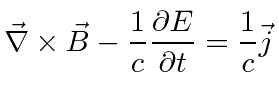## Rationalized Heaviside-Lorentz Units

The SI units are based on a unit of length of the order of human size originally related to the size of the earth, a unit of time approximately equal to the time between heartbeats, and a unit of mass related to the length unit and the mass of water. None of these depend on any even nearly fundamental physical quantities. Therefore many important physical equations end up with extra (needless) constants in them like. Even with the three basic units defined, we could have chosen the unit of charge correctly to makeandunnecessary but instead a very arbitrary choice was madeand the Ampere is defined by the current in parallel wires at one meter distance from each other that gives a force ofNewtons per meter. The Coulomb is set so that the Ampere is one Coulomb per second. With these choices SI units make Maxwell's equations and our filed theory look very messy.

Physicists have more often used CGS units in which the unit of charge and definition of the field units are set so thatandso they need not show up in the equations. The CGS units are not perfect, however, and we will want to change them slightly to make our theory of the Maxwell Field simple. The mistake made in defining CGS units was in removing thethat show up in Coulombs law. Coulombs law is not fundamental and thebelonged there.

We will correct this little mistake and move to Rationalized Heaviside-Lorentz Units by making a minor modification to the unit of charge and the units of fields. With this modification, our field theory will have few constants to carry around. As the name of the system of units suggests, the problem with CGS has been with. We don't need to change the centimeter, gram or second to fix the problem.

In Rationalized Heaviside-Lorentz units we decrease the field strength by a factor ofand increase the charges by the same factor, leaving the force unchanged.Its not a very big change but it would have been nice if Maxwell had started with this set of units. Of course the value ofcannot change, but, the formula for it does because we have redefined the charge.

Maxwell's Equations in CGS units areThe Lorentz Force isWhen we change to Rationalized Heaviside-Lorentz units, the equations becomeThat is, the equations remain the same except the factors ofin front of the source terms disappear. Of course, it would still be convenient to setsince this has been confusing us about 4D geometry andis the last unnecessary constant in Maxwell's equations. For our calculations, we can setany time we want unless we need answers in centimeters.

Jim Branson 2013-04-22